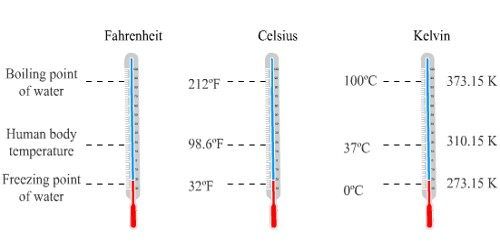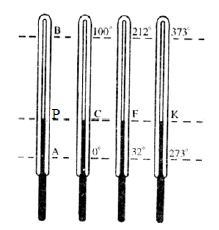# Establishing a Relation Among different Scales of Temperature

Generally, there are three common types of temperature units: Kelvin (K), Celsius (C) and Fahrenheit (F). Let A and B be the lower fixed point and upper fixed point of a thermometer respectively.Three more thermometers graduated in Celsius, Fahrenheit and Kelvin’s scale are kept side by side. The readings of the point P of the thermometer AB in the other three scales are C, F, and K respectively. Therefore the readings of distance PA in these three scales are C-0, F-32, and K-273 respectively. Since PA/BA is a constant it can be written as,

PA/BA = C-0 / 100-0 = F-32 / 212-32 = K-273 / 373-273

or, C/100 = F-32 / 180 = K-273 / 100

or, C/5 = F-32 / 9 = K-273 / 5

This equation indicates the relation between Celsius, Fahrenheit and Kelvin scale. The convenient relation between Celsius and Kelvin scale is — by adding 273 to Celsius scale reading the Kelvin scale reading can be obtained. For example, 1°C temperature = (1+273) K = 274 K temperature. But if the temperature difference is 1K, then it is equal to 1°C. The Kelvin scale is an absolute, thermodynamic scale with the two defining points absolute zero at 0 and the triple point of water at 273.16.

The Celsius temperature is defined to be accurately 273.15 less than the Kelvin temperature. The Fahrenheit scale is defined so that the Fahrenheit temperature is 32 more than 1.8 times the Celsius temperature.

Mostly, Kelvin and Celsius are used in thermal science-related calculations.

Now, Let’s Talk about relations –

Kelvin and Celsius: K = C + 273

Celsius and Fahrenheit: F = 9/5 (C) + 32

Let’s make it simple to remember and convert:

C/5 = (F-32)/9 = (K+273)/5.

In Summary: Quickly Convert Between Temperatures –

• Celsius to Fahrenheit: Multiply the temperature by 2 and then add 30
• Fahrenheit to Celsius: subtract 30 from the temp. and then divide by 2
• Kelvin to Fahrenheit: Subtract 273.15, multiply by 1.8, then add 32
• Fahrenheit to Kelvin: Subtract 32, multiply by 5, divide by 9, then add 273.15
• Kelvin to Celsius: Add 273
• Celsius to Kelvin: Subtract 273.